# Solving Equations of Motion for Systems of Particles with MATLAB (Part - 1) Civil Engineering (CE) Notes | EduRev

## Civil Engineering (CE) : Solving Equations of Motion for Systems of Particles with MATLAB (Part - 1) Civil Engineering (CE) Notes | EduRev

The document Solving Equations of Motion for Systems of Particles with MATLAB (Part - 1) Civil Engineering (CE) Notes | EduRev is a part of the Civil Engineering (CE) Course Introduction to Dynamics and Vibrations- Notes, Videos, MCQs.
All you need of Civil Engineering (CE) at this link: Civil Engineering (CE)

3.3 Deriving and solving equations of motion for systems of particles

We next turn to the more difficult problem of predicting the motion of a system that is subjected to a set of forces.

3.3.1 General procedure for deriving and solving equations of motion for systems of particles

It is very straightforward to analyze the motion of systems of particles. You should always use the following procedure

1. Introduce a set of variables that can describe the motion of the system. Don’t worry if this sounds vague – it will be clear what this means when we solve specific examples.
2. Write down the position vector of each particle in the system in terms of these variables
3. Differentiate the position vector(s), to calculate the velocity and acceleration of each particle in terms of your variables;
4. Draw a free body diagram showing the forces acting on each particle. You may need to introduce variables to describe reaction forces. Write down the resultant force vector. 5. Write down Newton’s law F = ma for each particle. This will generate up to 3 equations of motion (one for each vector component) for each particle.
6. If you wish, you can eliminate any unknown reaction forces from Newton’s laws. If you are trying to solve the equations by hand, you should always do this; of you are using MATLAB, it’s not usually necessary – you can have MATLAB calculate the reactions for you. The result will be a set of differential equations for the variables defined in step (1)
7. If you find you have fewer equations than unknown variables, you should look for any constraints that restrict the motion of the particles. The constraints must be expressed in terms of the unknown accelerations.
8. Identify the initial conditions for the variables defined in (1). These are usually the values of the unknown variables, their time derivatives, at time t=0. If you happen to know the values of the variables at some other instant in time, you can use that too. If you don’t know their values at all, you should just introduce new (unknown) variables to denote the initial conditions.
9. Solve the differential equations, subject to the initial conditions.

Steps (3) (6) and (8) can usually be done on the computer, so you don’t actually have to do much calculus or math.

Sometimes, you can avoid solving the equations of motion completely, by using conservation laws – conservation of energy, or conservation of momentum – to calculate quantities of interest. These shortcuts will be discussed in the next chapter.

3.2.2 Simple examples of equations of motion and their solutions

The general process described in the preceding section can be illustrated using simple examples. In this section, we derive equations of motion for a number of simple systems, and find their solutions.

The purpose of these examples is to illustrate the straightforward, step-by-step procedure for analyzing motion in a system. Although we solve several problems of practical interest, we will simply set up and solve the equations of motion with some arbitrary values for system parameter, and won’t attempt to explore their behavior in detail. More detailed discussions of the behavior of dynamical systems will follow in later chapters.

Example 1: Trajectory of a particle near the earth’s surface (no air resistance)

At time t=0, a projectile with mass m is launched from a position X0 = Xi + Yj + ZK with initial velocity vector V0 = Vxi +Vyj +Vzk. Calculate its trajectory as a function of time.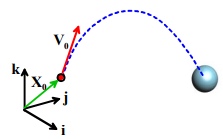1. Introduce variables to describe the motion: We can simply use the Cartesian coordinates of the particle (x(t), y(t), z(t))

2. Write down the position vector in terms of these variables: r = x(t)i + y(t) j + z(t)k

3. Differentiate the position vector with respect to time to find the acceleration. For this example, this is trivial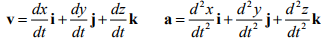4. Draw a free body diagram. The only force acting on the particle is gravity – the free body diagram is shown in the figure. The force vector follows as  F = -mgk.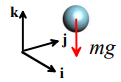5. Write down Newton’s laws of motion. This is easy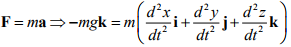The vector equation actually represents three separate differential equations of motion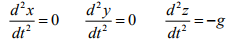6. Eliminate reactions – this is not needed in this example.

7. Identify initial conditions. The initial conditions were given in this problem – we have that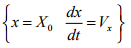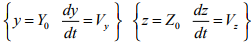8. Solve the equations of motion. In general we will use MAPLE or matlab to do the rather tedious algebra necessary to solve the equations of motion. Here, however, we will integrate the equations by hand, just to show that there is no magic in MAPLE.

The equations of motion are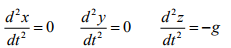It is a bit easier to see how to solve these if we define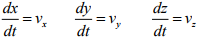The equation of motion can be re-written in terms of (vx,vy,vz) as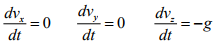We can separate variables and integrate, using the initial conditions as limits of integrationNow we can re-write the velocity components in terms of (x,y,z) as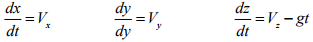Again, we can separate variables and integrate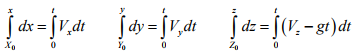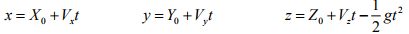so the position and velocity vectors are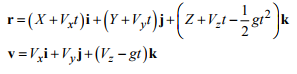Here’s how to integrate the equations of motion using Mupad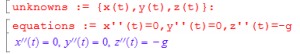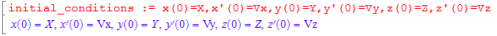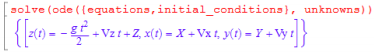Applications of trajectory problems: It is traditional in elementary physics and dynamics courses to solve vast numbers of problems involving particle trajectories. These invariably involve being given some information about the trajectory, which you must then use to work out something else. These problems are all somewhat tedious, but we will show a couple of examples to uphold the fine traditions of a 19th century education.

Estimate how far you could throw a stone from the top of the Kremlin palace.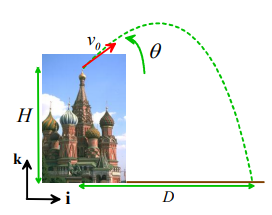Note that

1. The horizontal and vertical components of velocity at time t=0 follow as

Vx = v0 cosθ  Vy = 0 Vz = v0 sinθ

2. The components of the position of the particle at time t=0 are X = 0,Y = 0, Z = H

3. The trajectory of the particle follows as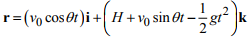4. When the particle hits the ground, its position vector is r = Di. This must be on the trajectory, so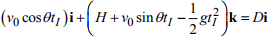where tI is the time of impact.

5. The two components of this vector equation gives us two equations for the two unknowns {tI, D} , which can be solved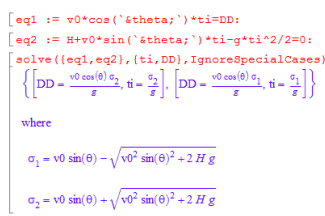The RootOf in MAPLE is scary – any time that MAPLE gives you a scary result, look in the help and see if there is a `convert’ function that might make it less scary.

For a rough estimate of the distance we can use the following numbers

1. Height of Kremlin palace – 71m
2. Throwing velocity – maybe 25mph? (pretty pathetic, I know - you can probably do better, especially if you are on the baseball team).
3. Throwing angle – 45 degrees. Substituting numbers gives 36m (118ft). If you want to go wild, you can maximize D with respect to θ , but this won’t improve your estimate much.

Silicon nanoparticles with radius 20nm are in thermal motion near a flat surface. At the surface, they have an average velocity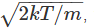where m is their mass, T is the temperature and k=1.3806503 � 10-23 is the Boltzmann constant. Estimate the maximum height above the surface that a typical particle can reach during its thermal motion, assuming that the only force acting on the particles is gravity1. The particle will reach its maximum height if it happens to be traveling vertically, and does not collide with any other particles.

2. At time t=0 such a particle has position X = 0, Y = 0, Z = 0 and velocity Vx = 0 Vy = 0 Vz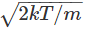3. For time t>0 the position vector of the particle follows as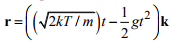Its velocity is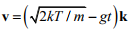4. When the particle reaches its maximum height, its velocity must be equal to zero (if you don’t see this by visualizing the motion of the particle, you can use the mathematical statement that if r = yk is a maximum, then  dr / dt = v = (dy / dt)k = 0).  Therefore, at the instant of maximum heigh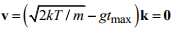5. This shows that the instant of max height occurs at time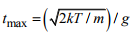6. Substituting this time back into the position vector shows that the position vector at max height is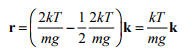7. Si has a density of about 2330 kg/m3. At room temperature (293K) we find that the distance is surprisingly large: 10mm or so. Gravity is a very weak force at the nano-scale – surface forces acting between the particles, and the particles and the surface, are much larger.

Example 2: Free vibration of a suspension system.

A vehicle suspension can be idealized as a mass m supported by a spring. The spring has stiffness k and un-stretched length L0 . To test the suspension, the vehicle is constrained to move vertically, as shown in the figure. It is set in motion by stretching the spring to a length L and then releasing it (from rest). Find an expression for the motion of the vehicle after it is released.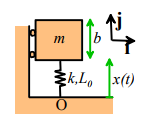As an aside, it is worth noting that a particle idealization is usually too crude to model a vehicle – a rigid body approximation is much better. In this case, however, we assume that the vehicle does not rotate. Under these conditions the equations of motion for a rigid body reduce to F = ma and M = 0, and we shall find that we can analyze the system as if it were a particle.

1. Introduce variables to describe the motion: The length of the spring xt() is a convenient way to describe motion.

2. Write down the position vector in terms of these variables: We can take the origin at O as shown in the figure. The position vector of the center of mass of the block is then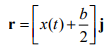3. Differentiate the position vector with respect to time to find the acceleration. For this example, this is trivial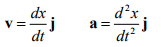4. Draw a free body diagram. The free body diagram is shown in the figure: the mass is subjected to the following forces

• Gravity, acting at the center of mass of the vehicle
• The force due to the spring
• Reaction forces at each of the rollers that force the vehicle to move vertically.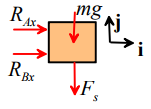Recall the spring force law, which says that the forces exerted by a spring act parallel to its length, tend to shorten the spring, and are proportional to the difference between the length of the spring and its unstretched length.

5. Write down Newton’s laws of motion. This is easy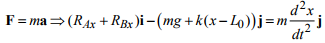The i and j components give two scalar equations of motion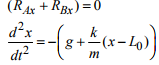6. Eliminate reactions – this is not needed in this example.

7. Identify initial conditions. The initial conditions were given in this problem – at time t=0, we know that x = Land dx / dt = 0

8. Solve the equations of motion. Again, we will first integrate the equations of motion by hand, and then repeat the calculation with MAPLE. The equation of motion is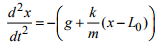We can re-write this in terms of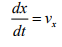This givesWe can separate variables and integrate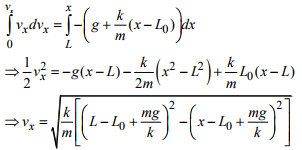Don’t worry if the last line looks mysterious – writing the solution in this form just makes the algebra a bit simpler. We can now integrate the velocity to find x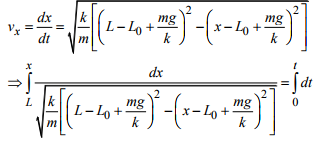The integral on the left can be evaluated using the substitution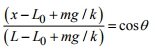so that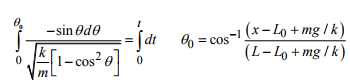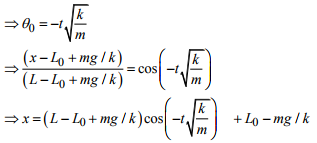Here’s the MAPLE solution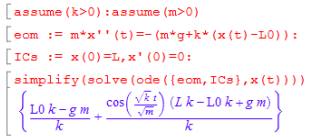Note that it’s important to include the assume() statements, otherwise Mupad gives the solution in the form of an exponential of a complex number. The solution in this other form is also correct, but is difficult to visualize.

The solution is plotted in the figure. The behavior of vibrating systems will be discussed in more detail later in this course, but it is worth noting some features of the solution: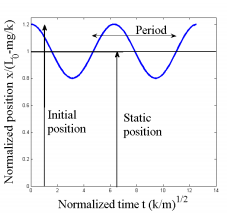1. The average position of the mass is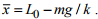Here, mg/k is the static deflection of the spring i.e. the deflection of the spring due to the weight of the vehicle (without motion). This means that the car vibrates symmetrically about its static deflection.

2. The amplitude of vibration is L-L0+mg/k . This corresponds to the distance of the mass above its average position at time t=0.

3. The period of oscillation (the time taken for one complete cycle of vibration) is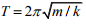4. The frequency of oscillation (the number of cycles per second)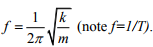Frequency is also sometimes quoted as angular frequency, which is related to f by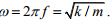Angular frequency is in radians per second.

An interesting feature of these results is that the static deflection is related to the frequency of oscillation - so if you measure the static deflection δ = mg k/ , you can calculate the (angular) vibration frequency as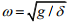Offer running on EduRev: Apply code STAYHOME200 to get INR 200 off on our premium plan EduRev Infinity!

## Introduction to Dynamics and Vibrations- Notes, Videos, MCQs

20 videos|53 docs

,

,

,

,

,

,

,

,

,

,

,

,

,

,

,

,

,

,

,

,

,

;